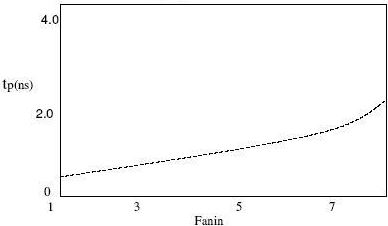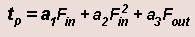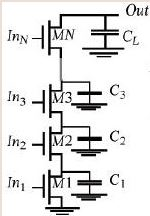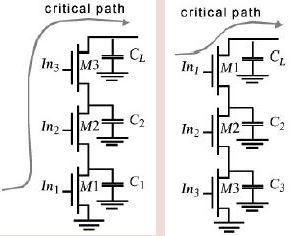Courses

# Dependence of Propagation Delay on Fan-in and Fan-out Electrical Engineering (EE) Notes | EduRev

## VLSI System Design

Created by: Machine Experts

## Electrical Engineering (EE) : Dependence of Propagation Delay on Fan-in and Fan-out Electrical Engineering (EE) Notes | EduRev

The document Dependence of Propagation Delay on Fan-in and Fan-out Electrical Engineering (EE) Notes | EduRev is a part of the Electrical Engineering (EE) Course VLSI System Design.
All you need of Electrical Engineering (EE) at this link: Electrical Engineering (EE)

Objectives

In this lecture you will learn the following

• Motivation
• Design Techniques for large Fan-in

18.1 Motivation

First we will show you how the fan-in and fan-out depends on propagation delay and then we will analyze how to make Fan-in large.

The propagation delay of a CMOS gate deteriorates rapidly as a function of the fan-in . firstly the large number of transistor (2N) increases the overall capacitance of the gate. Secondly a series connection of transistor either in the PUN or PDN slows the gate as well, because the effective (dis)charging resistance is increased .

Fan-out has a lager impact on the gate delay in complementary CMOS than some other logic states. In complementary circuit
style, each input connects to both an NMOS and a PMOS device and presents a load to the driving gate equal to the sum of the gates capacitances.Fig 18.1: Dependence of Propagation delay on Fan-in

Thus we can approximate the influence of fan in and fan-out on propagation delay in complementary CMOS gate as :Where a1, a2 and a3 are weighing factor which are a function of technology

18. 2 Design techniques for large fan in

1. Transistor Sizing: Increasing the transistor sizes increases the available (dis)charging current. But widening the transistor results in large parasitic capacitor. This does not only affect the propagation delay of the gate but also present a larger load to the preceding gate.

2. Progressive Transistor Sizing: Usually we assume that all the intrinsic capacitances, in a series connected array of transistors, can be lumped into a single load capacitance CL and no capacitance is present at the internal nodes of network.Fig 18.21: Illustration of Progressive Transistor Sizing

Under these assumptions making all transistors in a series chain equal in size makes sense. This model is an over-simplification, and become more and more incorrect for increasing fan in. referring to the circuit below we can see that the capacitor associated with the transistor as we go down the chain increases and so the transistor has to discharge an increasing current as we go down the chain. While transistor MN has to conduct the discharge current only of load capacitance CL. M1has to carry the discharge current from the total capacitance Ctot =C1+ C2 + ....+CL, which is substantially larger. Consequently a progressive scaling of the transistors is beneficial. M1>M2>M3>....>MN. This technique has for instance proven to be advantageous in the decoders of memories where gates with large fan in are common. The effect of progressive sizing can be
understood by the circuit in fig 18.21.

Spice simulation Example:

Taking CL=15 fF; N =5; C1=C2=C3=C4=10fF.

When all transistors are of minimum size SPICE predicts a propagation delay of 1.1nsecs. The transistors M5 toM1 are then made progressively wider in such a way that the width of the transistor is proportional to the total capacitor it has to discharge. M5 is of minimum size, WM4=WM5(CLC4)/CL, WM3=WM5(CL+C3+C4)/CL and so on. The resulting circuit has tpHL of 0.81nsecs or a reduction of 26.5%.

3. Transistor Ordering: Some signals in complex combinational logic blocks might be more critical than others .no all inputs ofFig18.21: Two examples            circuits for critical path

a gate arrive at the same time (may be due to propagation delays of the preceding blocks). An input signal to a gate is called critical if it is the last signal of all input to assume a stable value. The path through the logic which determines the ultimate speed of the structures is called the critical path. Putting the critical path transistor closer to the output of the gate can result in a speed up. Referring to the figure given below signal In1 is assumed to be the critical signal. Suppose we assume signal In2 and In3 are high and In1 undergoes a 0 to transition. Assume also that CL is initially charged high in 1st case no path to ground exists until M1 is turned on .the delay between the arrival of In1 and the output is therefore determined by the time it takes to discharge CL + C1 + C2. In the 2nd case C1 and C2 are already discharged when In1 changes. Only CL has to be discharged, resulting in a faster response time.

Using SPICE thetPHL for a 4-input NAND gate was calculated.

With the critical input connected to the bottommost transistor the tpd =717ns and when connected to the uppermost transistor tpd= 607 ns, an improvement of 15%.

Offer running on EduRev: Apply code STAYHOME200 to get INR 200 off on our premium plan EduRev Infinity!

35 docs|28 tests

,

,

,

,

,

,

,

,

,

,

,

,

,

,

,

,

,

,

,

,

,

;